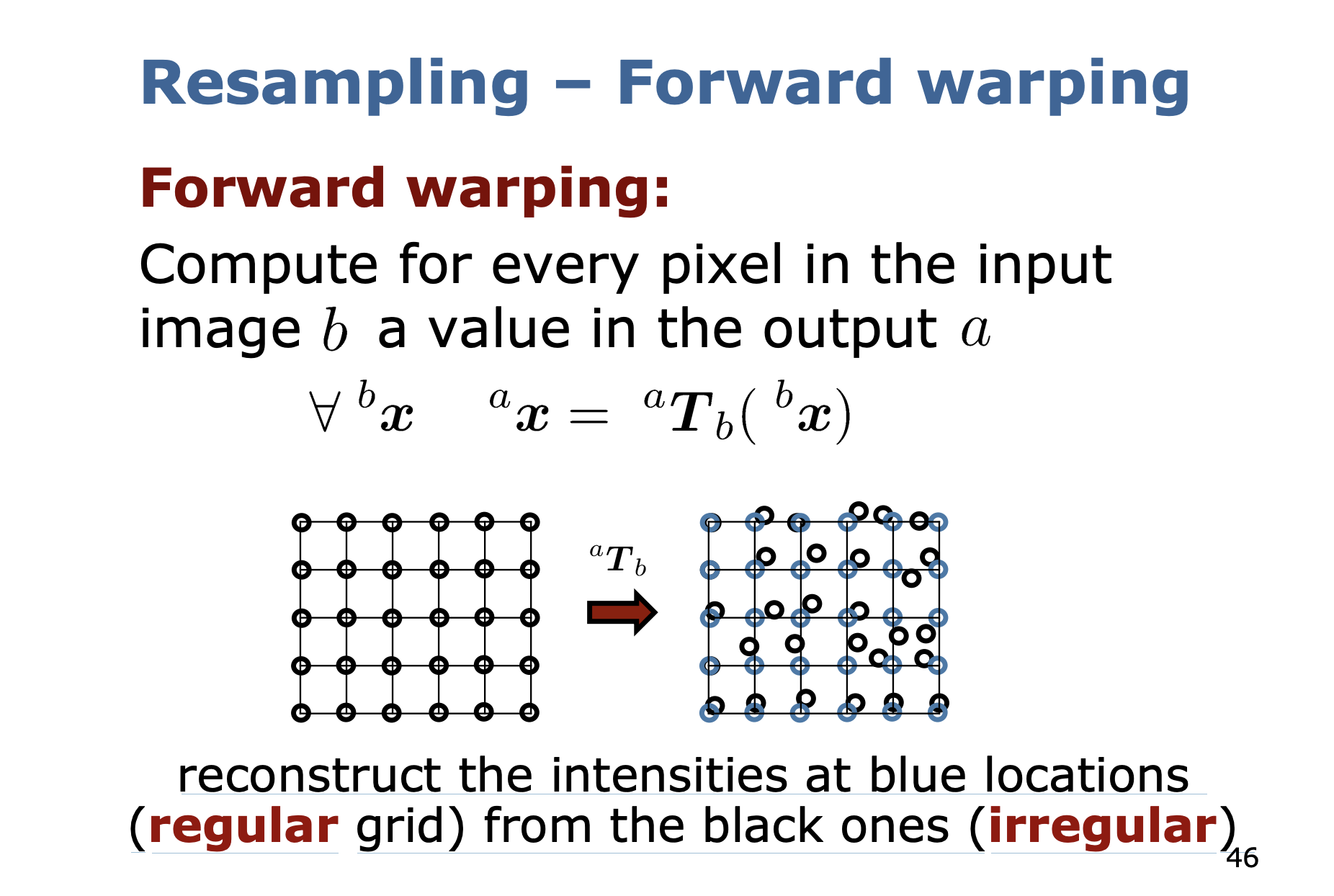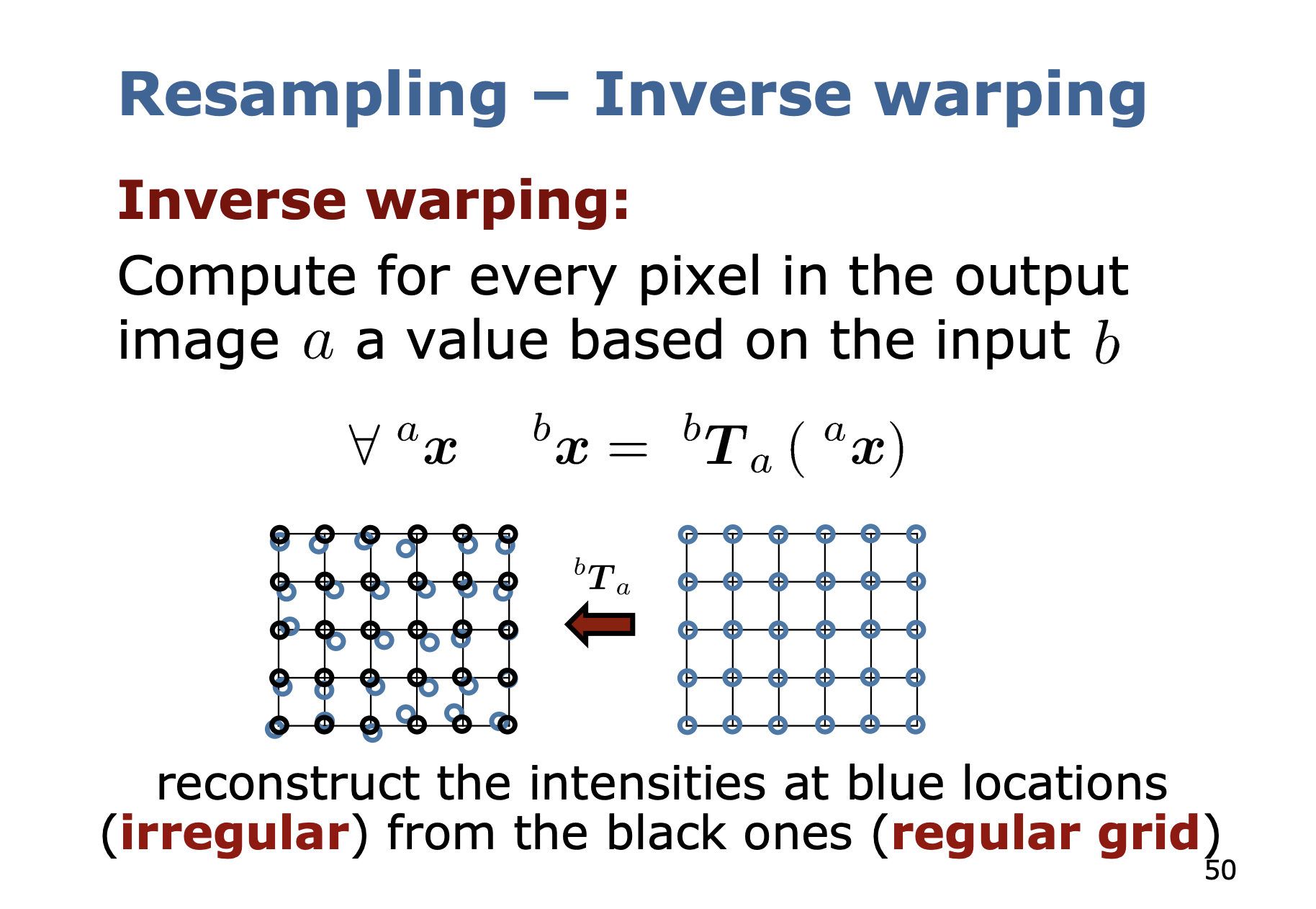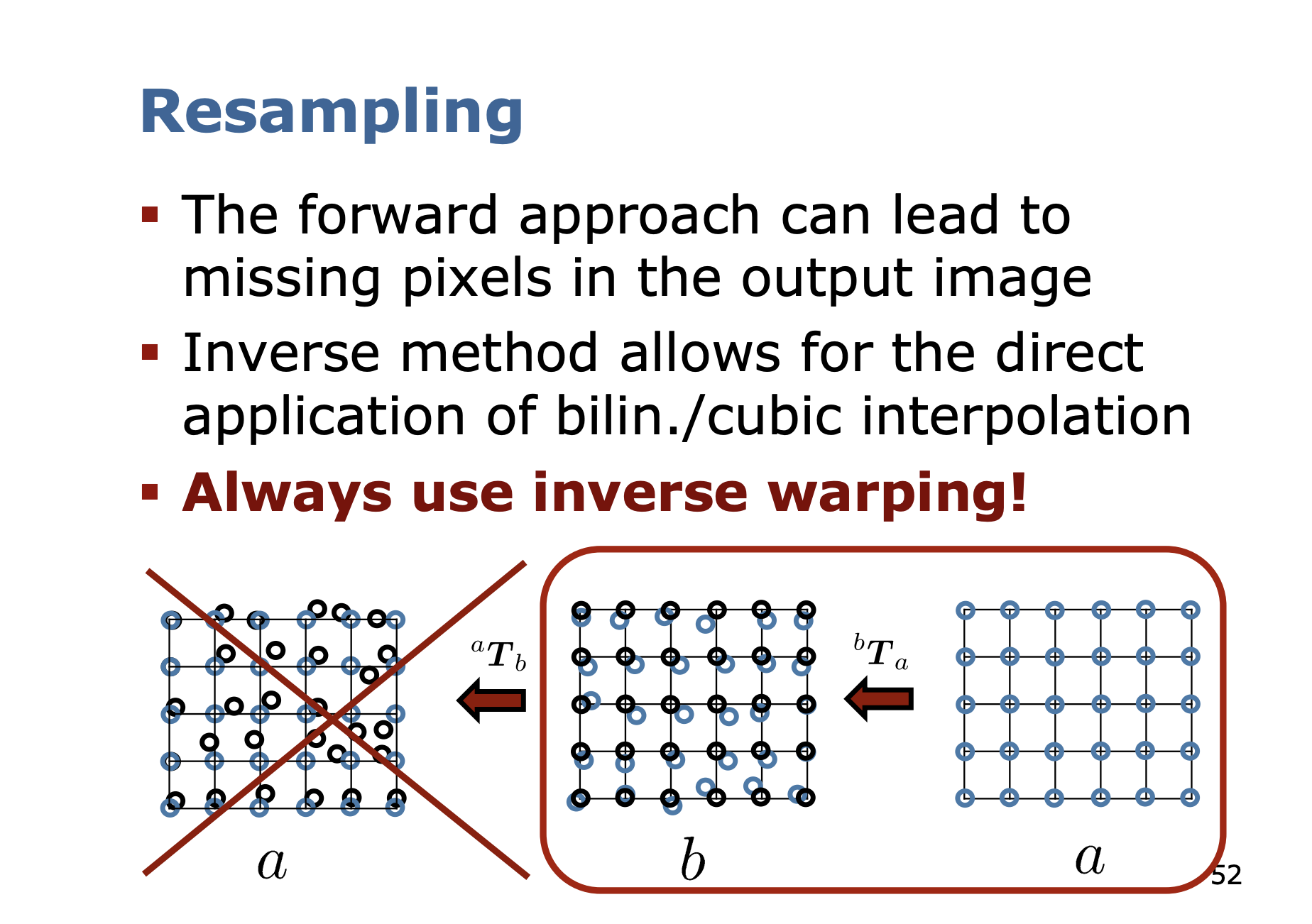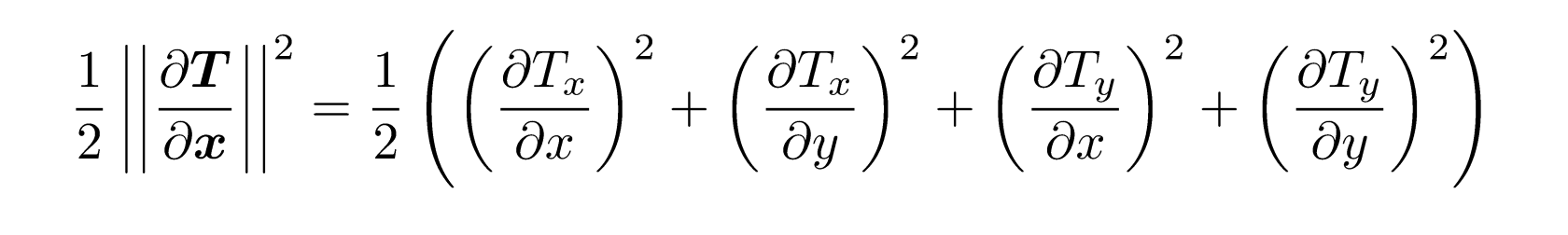Image Interpolation

# Resampling §

Notes from Cyrill Stachniss.

Resampling

Resampling refers to the process of changing the dimensions or geometry of an image.

Resampling is performing a discretization and quantization.

When is resampling actually used?

• Image zooming
• Rotation of image
• Geometry tranformations (skewing, warping, perspective transformions)

What is the problem that resampling is solving?

These transformations that we are applying on images lead to non-integer coordinates.

To assign intensity values from the input to the output images, we need to interpolate.

Resampling - Forward Warping

• Compute for every pixel in the input image a value in the outputInverse WarpingForward or inverse warping?

Always use inverse warping!

• The forward approach can lead to missing pixels in the output image
• Inverse method allows for the direct application of bilin./cubic interpolation

You can get more complex warping behavior##### Image Half-Sizing §

If you are taking half the size, you are neglecting information.

Use the matrix to remap the pixel positions.

Through simple subsampling, we get aliasing artifacts.

Solution: Apply a Binomial Filter before subsampling.

Why is smoothing step needed?

• Simple subsampling results in aliasing and loosing details
• Smoothing combines pixel information from neighbouring pixels

This is the kernel that was used

How much smoothing is needed?

• Depends on the kernel
• Depends on the width of the kernel
• Depends on the scale of the transformation

Width of a kernel is given by its standard deviation.

For the box filter

For the binomial filter

Scale of a Transformation

where you have the following expansionFor the Gaussian filter

Consider the Scale for Resampling

• : Image becomes smaller
• Recommendation:
• : Same scale
• Use bilinear interpolation or bicubic for high quality results
• : Image becomes larger
• Use bicubic interpolation

What is the point of same scale case?

think about the rotation, or some shift that you want to take into account.